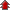Home Board Paper Solutions Text Book Solutions Articles NCERT Exemplar Solutions

# The s Block Elements### Exercise 1

•  Q1 What are the common physical and chemical features of alkali metals? Q2 Discuss the general characteristics and gradation in properties of alkaline earth metals. Q3 Why are alkali metals not found in nature? Q4 Find the oxidation state of sodium in Na2O2. Q5 Explain why is sodium less reactive than potassium? Q6 Compare the alkali metals and alkaline earth metals with respect to (i) ionization enthalpy (ii) basicity of oxides and (iii) solubility of hydroxides.">Compare the alkali metals and alkaline earth metals with respect to (i) ionization enthalpy (ii) basicity of oxides and (iii) solubility of hydroxides. Q7 In what ways lithium shows similarities to magnesium in its chemical behaviour? Q8 Explain why alkali and alkaline earth metals cannot be obtained by chemical reduction methods?">Explain why alkali and alkaline earth metals cannot be obtained by chemical reduction methods? Q9 Why are potassium and cesium, rather than lithium used in photoelectric cells? Q10 When an alkali metal dissolves in liquid ammonia the solution can acquire different colours. Explain the reasons for this type of colour change.">When an alkali metal dissolves in liquid ammonia the solution can acquire different colours. Explain the reasons for this type of colour change. Q11 Beryllium and magnesium do not give colour to flame whereas other alkaline earth metals do so. Why? Q12 Discuss the various reactions that occur in the Solvay process. Q13 Potassium carbonate cannot be prepared by Solvay process. Why? Q14 Why is Li2CO3 decomposed at a lower temperature whereas Na2CO3 at higher temperature? Q15 Compare the solubility and thermal stability of the following compounds of the alkali metals with those of the alkaline earth metals. (a) Nitrates (b) Carbonates (c) Sulphates.">Compare the solubility and thermal stability of the following compounds of the alkali metals with those of the alkaline earth metals. (a) Nitrates (b) Carbonates (c) Sulphates. Q16 Starting with sodium chloride how would you proceed to prepare (i) sodium metal (ii) sodium hydroxide (iii) sodium peroxide (iv) sodium carbonate? Q17 What happens when (i) magnesium is burnt in air (ii) quick lime is heated with silica (iii) chlorine reacts with slaked lime (iv) calcium nitrate is heated ?">What happens when (i) magnesium is burnt in air (ii) quick lime is heated with silica (iii) chlorine reacts with slaked lime (iv) calcium nitrate is heated ? Q18 Describe two important uses of each of the following: (i) caustic soda (ii) sodium carbonate (iii) quicklime. Q19 Draw the structure of (i) BeCl2 (vapour) (ii) BeCl2 (solid). Q20 The hydroxides and carbonates of sodium and potassium are easily soluble in water while the corresponding salts of magnesium and calcium are sparingly soluble in water. Explain.">The hydroxides and carbonates of sodium and potassium are easily soluble in water while the corresponding salts of magnesium and calcium are sparingly soluble in water. Explain. Q21 Describe the importance of the following: (i) limestone (ii) cement (iii) plaster of paris. Q22 Why are lithium salts commonly hydrated and those of the other alkali metal ions usually anhydrous?">Why are lithium salts commonly hydrated and those of the other alkali metal ions usually anhydrous? Q23 Why is LiF almost insoluble in water whereas LiCl soluble not only in water but also in acetone? Q24 Explain the significance of sodium, potassium, magnesium and calcium inbiological fluids.">Explain the significance of sodium, potassium, magnesium and calcium inbiological fluids. Q25 What happens when (i) sodium metal is dropped in water ? (ii) sodium metal is heated in free supply of air ? (iii) sodium peroxide dissolves in water ? Q26 Comment on each of the following observations: (a) The mobilities of the alkali metal ions in aqueous solution are Li+ <  Na+ <  K+ <  Rb+ < Cs+ (b) Lithium is the only alkali metal to form a nitride directly. (c) E° for M2+(aq) (where M = Ca, Sr or Ba) is nearly constant. Q27 State as to why (a) a solution of Na2CO3 is alkaline ? (b) alkali metals are prepared by electrolysis of their fused chlorides ? (c) sodium is found to be more useful than potassium ? Q28 Write balanced equations for reactions between (a) Na2O2and water (b) KO2 and water (c) Na2O and CO2 Q29 How would you explain the following observations? (i) BeO is almost insoluble but BeSO4 in soluble in water, (ii) BaO is soluble but BaSO4 is insoluble in water, (iii) LiI is more soluble than KI in ethanol. Q30 Which of the alkali metal is having least melting point? (a) Na (b) K (c) Rb (d) Cs Q31 Which one of the following alkali metals gives hydrated salts? (a) Li (b) Na (c) K (d) Cs Q32 Which one of the alkaline earth metal carbonates is thermally the most stable? (a) MgCO3 (b) CaCO3 (c) SrCO3 (d) BaCO3